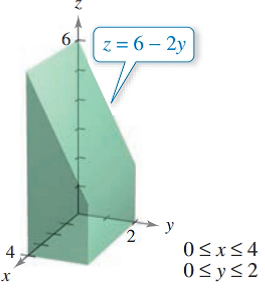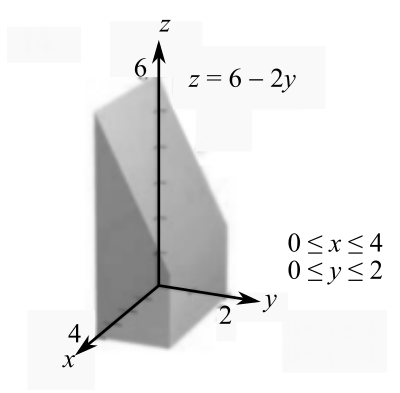Chapter 7.9, Problem 14E### Calculus: An Applied Approach (Min...

10th Edition
Ron Larson
ISBN: 9781305860919

#### Solutions

Chapter
Section### Calculus: An Applied Approach (Min...

10th Edition
Ron Larson
ISBN: 9781305860919
Textbook Problem
1 views

# Finding the Volume of a Solid Region In Exercises 13-20, use a double integral to find the volume of the solid region.To determine

To calculate: The volume of the solid region bounded by z=62y, 0x4 and 0y2.Explanation

Given Information:

The provided function is z=62y and the range of x and y are 0x4, 0y2.

And, the provided figure is,

Formula used:

Step 1: First decide the order of integration,

If V=aby=f(x)x=g(x)f(x,y)dydx then take strip parallel to the y-axis.

If V=aby=f(x)x=g(y)f(x,y)dydx then take strip parallel to the x-axis.

Step 2: Integrate the function f(x,y).

If the order of integration dydx then integrate function first with respect to y and x constant

apply the limit. The integrate with respect x.

Step 3: Substitute the limit of x and get the volume of a given function

### Still sussing out bartleby?

Check out a sample textbook solution.

See a sample solution

#### The Solution to Your Study Problems

Bartleby provides explanations to thousands of textbook problems written by our experts, many with advanced degrees!

Get Started

#### What are the two requirements for a random sample?

Statistics for The Behavioral Sciences (MindTap Course List)

#### In problems 1-16, solve each equation. 19. Solve the Fractional equation

Mathematical Applications for the Management, Life, and Social Sciences

#### For

Study Guide for Stewart's Multivariable Calculus, 8th

#### Define a construct and explain the role that constructs play in theories.

Research Methods for the Behavioral Sciences (MindTap Course List)